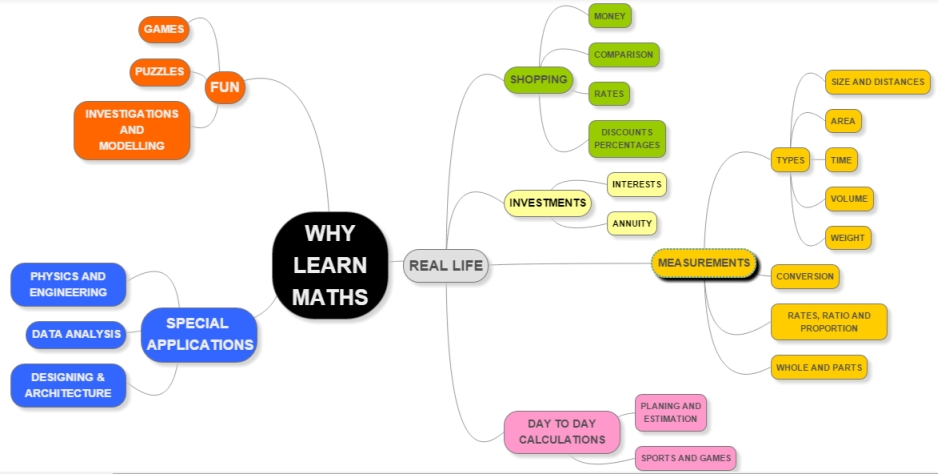# Flocci - Maths - Why Learn Maths

## Primary tabsIf one does not like maths or finds it difficult - one rejects maths or oneself :-(
When one becomes aware of different roles of maths - then it allows to not only see maths in a new way - but also to choose what one wants to explore.

Here is an attempt to share three roles of maths.

Why Maths:

Real life - What one needs to live a regular life - the kind of maths most people use. We normally use basic computation skills and measurements in our day to day life.

Math for fun - Anybody who has enjoyed Puzzles, maths games (say monopoly), etc - knows maths can be fun. Also for some of us exploring mathematical patterns, investigations etc can also be fun. This is for people who want to explore maths as art.

Maths used for special application  - One likes rockets and want to launch but do not know what would be trajectory. This application needs maths. So maths is a support for many specialized applications like physics, chemistry, financial analysis, etc. So one may need to explore maths because of what one wants to do - like a fighter pilot does!

Below find activities for each of these roles that can help children appreciate them:

Activity 1 - For Maths in Real Life
Take two bottles of Shampoo or any other packaged stuff - which has two different volume or weight and different price. Find out which one is costly / cheaper. Allow usage of calculator.

Activity 2 - For Maths for FUN
If we were 3 people in how many different ways we can stand in a line. If we were 4 in how many ways , then for 5 ... and so on - can you find a formula to find this for any number of people.

Alternatively you can flip a coin and guess what is the chance that you will get heads (do it 10 time and see what result you get. Do it 20 times and so on.0

Activity 3 - For Maths in Special Application (Physics)

1. Take a scale and balance it on top of a pencil - like a balanced see-saw
2. Now take a Rs1 coin and put it on the left end of the scale. What happens?
3. Now take two similar Rs1 coins and see where can you put these on the other side to be able to balance the scale again?
4. Play around with more coins on either side.
5. What is the mathematical relationship of left hand side to right hand side?
6. If require see animated demo here
7. STORE - Algerbra in real life -

### Activity 1 : Math for real

Activity 1 : Math for real life

Activity 2 ; Math for Fun

Children were told the activity and they deccided to stand in ascending decending order of there heghts. So all seven tried standing in a line in vertical and horizontal way.
Then they were trying to stand in a line according to their weight. So each one of them weighed themselves on a weighing machine. and made a line by standing from the lower weight to the higher weight.
Then they decided to stand according to their ages.  So they played around making lines in many different ways.

Activity 3 ; Math for Application

The children were told the activity. The children go their pencils and scales and started to balnce the scale on the pencil. Then they were given coins. The kids grouped themselves in pairs and one was tried to balance the scale on the pencil, while the other was trying to place the coins one at a time and sometimes both at the same time.

The activities were good to explore the different elements of "Why Learn Math". After the activities, when the children were asked, why we need to learn math, few replied to USE and few said, it was FUN doing it.